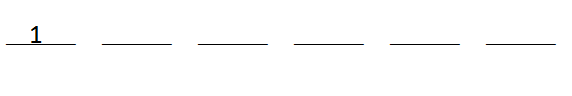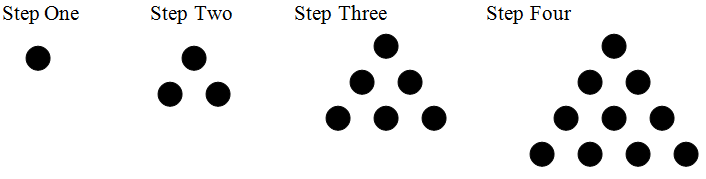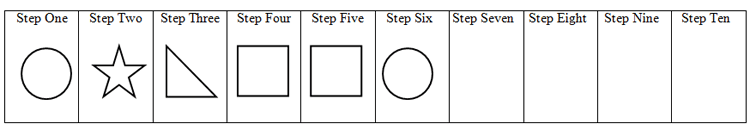4.OA.C.5 - Generate and Analyze Patterns Printable Tasks Worksheet

Name_______________________________________

Task #1 Multiply by 6

1. Beginning with 1, complete the following sequence of numbers by multiplying the previous number by 6.1. What do you notice about the numbers in the pattern?

1. If the pattern continues, what digit will be in the ones place of the seventh number?

Name______________________________________________

Task #2 Dot Patterns

Examine the dot pattern below. For each step, a new row of dots is added to the bottom. In step one, one new dot is added. In step two, two new dots are added. In step three, three new dots are added. Assuming this pattern continues, find the total number of dots in each figure through step ten.Step # 1 2 3 4 5 6 7 8 9 10 Total number of Dots 1 3 6 10 - - - - - -

What patterns do you notice when looking at the total number of dots at each step?

Name ________________________________________

Task #3 Football Cards

Tony collects football cards. He started his collection with only six cards. Football cards come in packs of ten. If we let x represent the number of packs he purchases, you could use the rule 10x + 6 to determine how many cards he would have for any given number of packs he purchases.

Complete the table below by following the rule:

 Number of Packs Purchased 10x + 6 1 2 3 4 5 10 23 46 64 100

What do you notice about the numbers you inputted in the

Name ______________________________________________

Task #4 Shape PatternsThe pattern above is formed by repeating the first five figures in the pattern: circle, star, right triangle, square, square. Can you draw the next four shapes in the pattern?

Without drawing the shapes, can you tell what the 51st shape in the pattern will be?

How did you determine what the 51st